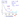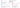## Concise.org

#### Testing convergence of gradient descent

Make a plot with number of iterations on the x-axis. Now plot the cost function, $J(θ)$ over the number of iterations of gradient descent.

Ideally, $J(\theta)$ should decrease after every iteration.Number of iterations taken by gradient descent to converge can vary a lot depending for different cases.

Example automatic convergence test:
Declare convergence if $J(\theta)$ decereases by less than $10^{-3} (\epsilon)$ in one iteration.

However, in practice choosing a particular threshold $(\epsilon)$ value can be pretty difficult. So, in order to test convergence of gradient descent, graphs like above can be more effective.

#### Debugging: How to make sure gradient descent is working correctlyIf J(θ) ever increases, then you probably need to decrease $\alpha$.

NOTE:
It has been proven that if learning rate $\alpha$ is sufficiently small, $J(\theta)$ should decrease on every iteration.

#### How to choose learning rate $\alpha$

Recall that:

• If $\alpha$ is too small:
• slow convergence
• If $\alpha$ is too large:
• $J(\theta)$ may not decrease on every iteration, and thus may not converge
• may even diverge
• sometimes, slow convergence also possible

In order to choose suitable $\alpha$, try a range of values and corresponding to each value of $\alpha$, plot the values of $J(\theta)$ as a function of number of iterations. Then, choose an $\alpha$ which causes $J(\theta)$ to decrease rapidly.

For trying a range of values of $\alpha$, you can choose factors of 10 or 3, for example.
E.g., ..., 0.001, 0.003, 0.01, 0.03, 0.1, 0.3, 1, ...